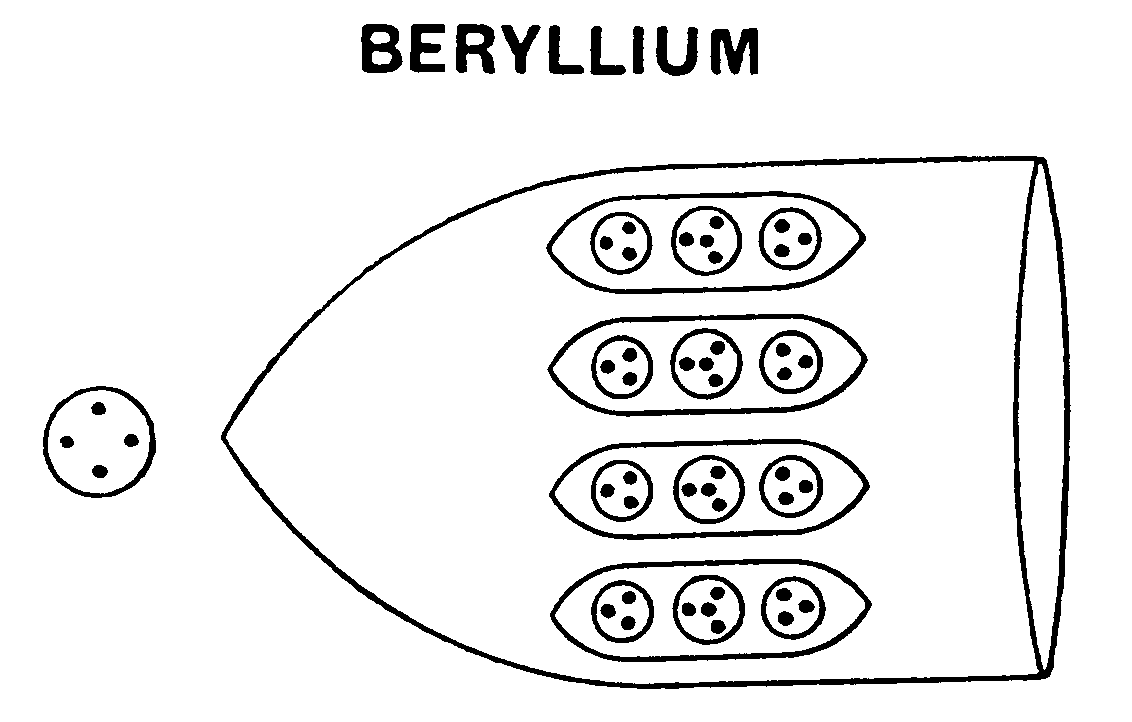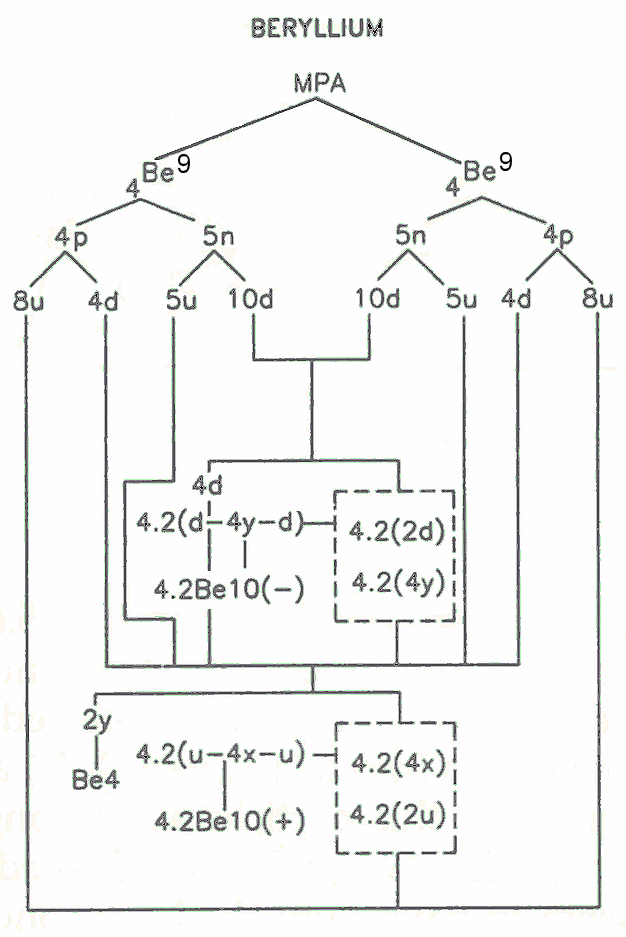Beryllium MPA

The MPA of beryllium is a tetrahedral array of four funnels radiating from a central globe. A funnel contains four ovoids (Be10), each enclosing two H3 triplets and one quartet of UPAs. The globe contains a whirling cross of four UPAs (Be4).

Beryllium MPA = Be4 + 4(4Be10).Tetrahedral array of four funnels in the beryllium MPA.                                                                A funnel and the central globe.

The MPA is formed from two Be9 nuclei, which provide 162 subquarks — two fewer than the number of UPAs. Because of the similarity of the four funnels, the predicted error of observation must reside in the central Be4 globe, which is predicted to contain two UPAs, as a (−) duad (Y-Y). The disintegration diagram shown below indicates that it breaks up into two (0) duads (X-Y), which, too, disagrees with prediction. Remarkably, however, the disintegration diagram of beryllium published in the 1st edition of OccultChemistry shows the Be4 group breaking up into a (+) duad (X-X) and a (−) duad (Y-Y), the latter agreeing with prediction. Presumably, this particle was wrongly observed on two separate occasions, the later event occurring after 1908, when the 1st edition was published, although Besant & Leadbeater do not seem to have published a second description of the beryllium MPA, as they did for some other elements. Two types of Be10 groups are predicted, one the mirror state of the other: the Be10(+) is a bound state of two u quarks and a bound state of four X subquarks, tetrahedrally arranged; the Be10(−) is a bound state of four Y subquarks, similarly arranged:

Be10(+) = 8X + 2Y = u + 4X + u

and

Be10(−) = Be10′ = 8Y + 2X = d + 4Y + d.

These mirror states have opposite electric polarity because they consist of counterpart mirror particles whose charges are of opposite sign. Two Be10(+) groups and two Be10(−) groups are in each funnel. The disintegration diagram confirms this because it shows that a funnel releases at the E3 stage two (+) Be10 groups and two (−) Be10 groups. In the former, the UPAs point outwards, in the latter they point inwards, a schematic convention that Besant & Leadbeater used always to indicate "positivity" and "negativity" of particles. Furthermore, the Be10(+) contains two (+) triplets (u quarks) and a (+) quartet of UPAs, which many disintegration diagrams in Occult Chemistry reveal can be further broken up into two (+) duads (X-X), confirming the composition of the Be10(+) given above, whilst the Be10(−) contains two (−) triplets (d quarks) and a (−) quartet of UPAs, which many disintegration diagrams indicate can be further broken up into two (−) duads (Y-Y), also in agreement with the composition of the Be10(−) given above. Below is shown the subquark composition of the Be10 groups in a funnel:Home